# Shell Scripting Part V: Functions in Bash

Hi! Welcome to HowToForge's shell scripting tutorial series. If you want to read the previous releases of the tutorial feel free to click here for part1, part2, part3 and part4 of the tutorial. In this part, you will learn how to efficiently structure your scripts by creating functions. By the end of this tutorial, you will be able to know how to create functions in the Linux Bash Shell, pass parameters to your functions and return some values from a function to your main code. Let's get started!

## Introduction

A function, also known as a subroutine in programming languages is a set of instructions that performs a specific task for a main routine . It allows programmers to break a complicated and lengthy code to small sections which can be called whenever needed. Each function needs to be called by a main routine in order to run, thus, it is isolated with other parts of your code and this creates an easy way of code testing. Additionally, functions can be called anytime and repeatedly, this allows you reuse, optimize and minimize your codes. Like most programming languages, the bash shell also supports functions.

### General Syntax:

1. Syntax 1:
``function function_name{    ##### set of commands}``
2. Syntax 2:
``function_name(){    #### set of commands}``

## Creating Functions

The bash supports two structures for functions. In using the first syntax, you have to use the keyword function, followed by your function name and open and close parentheses and curly braces to separate the contents of your functions to your main routine. You will find this syntax familiar if you have a background in PHP because functions in PHP are declared in the same way. The other syntax only consists of a function name, open and close parentheses and curly braces.

``#!/bin/bashmyfunction(){    echo "My function works!"}myfunction``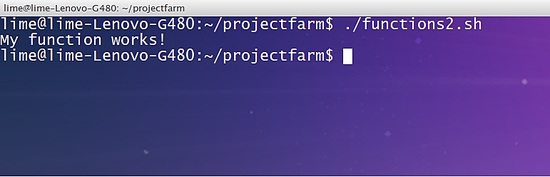I have used the second syntax in our example. After creating the function myfunction, it was then invoked by calling its function name to our main routine. The main routine will be anywhere in our script that was not defined as part of our function.

Now let's rearrange our code to test whether functions can be declared anywhere in our script. Consider the code below:

``#!/bin/bashecho "testing my function"myfunctionmyfunction(){    echo "My function works!"}``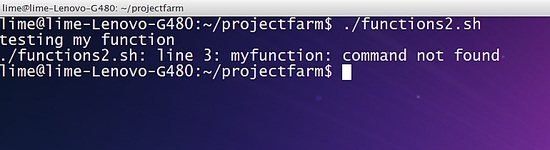The line 3 in the above code returns a command not found error. This only means that:

``The function only works if it is declared before your main routine. The interpreter will return an error if you have declared your function after your main routine.``

## Restructuring codes using functions

One of the best features of functions is being able to reuse codes. When a procedure requires repeatedly executing commands but could not be structured using looping statements then a function can be a solution.

For example, consider the code below:

``#!/bin/bashwhile(true)do    clear    printf "Choose from the following operations: \n"    printf "[a]ddition\n[b]Subtraction\n[c]Multiplication\n[d]Division\n"    printf "################################\n"    read -p "Your choice: " choice    case \$choice in    [aA])        read -p "Enter first integer: " int1        read -p "Enter second integer: " int2        res=\$((int1+int2))     ;;    [bB])        read -p "Enter first integer: " int1        read -p "Enter second integer: " int2    res=\$((int1-int2))     ;;    [cC])        read -p "Enter first integer: " int1        read -p "Enter second integer: " int2        res=\$((int1*int2))     ;;    [dD])        read -p "Enter first integer: " int1        read -p "Enter second integer: " int2        res=\$((int1/int2))     ;;    *)        res=0        echo "wrong choice!"    esac    echo "The result is: " \$res    read -p "Do you wish to continue? [y]es or [n]o: " ans    if [ \$ans == 'n' ]        then         echo "Exiting the script. Have a nice day!"        break    else        continue    fidone``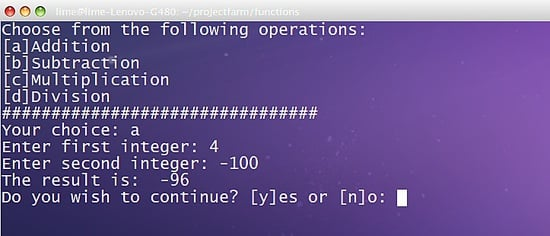The script is running well, however, notice that the lines for accepting inputs are repeatedly done in each pattern in our switch statement.

``#!/bin/bashinputs(){     read -p "Enter first integer: " int1    read -p "Enter second integer: " int2}exitPrompt(){    read -p "Do you wish to continue? [y]es or [n]o: " ans    if [ \$ans == 'n' ]    then        echo "Exiting the script. Have a nice day!"        break    else        continue    fi}while(true)    do    clear    printf "Choose from the following operations: \n"    printf "[a]Addition\n[b]Subtraction\n[c]Multiplication\n[d]Division\n"    printf "################################\n"    read -p "Your choice: " choice    case \$choice in    [aA])        inputs        res=\$((int1+int2))    ;;    [bB])        inputs        res=\$((int1-int2))    ;;    [cC])        inputs        res=\$((int1*int2))    ;;    [dD])        inputs        res=\$((int1/int2))    ;;    *)        res=0        echo "wrong choice!"    esac    echo "The result is: " \$res    exitPromptdone``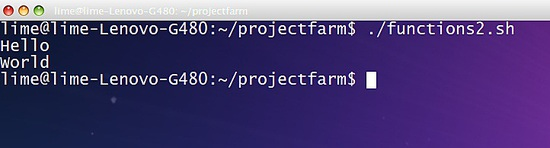We improved our code by creating subsections inputs and exitPrompt. It works exactly the same with our previous code, however, our current code is easier to troubleshoot because it is structured properly.

## Passing parameters on functions

Like most of the programming languages, you can pass parameters and process those data in functions in bash. The code below shows the procedure on how to pass values in shell scripting:

``#!/bin/bashmyfunction(){    echo \$1    echo \$2}myfunction "Hello" "World"``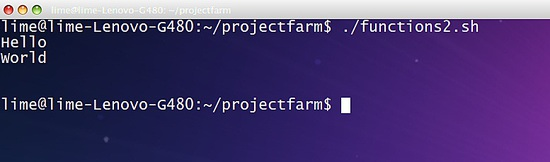Notice in our example, we added the values "Hello" and "World" after we called the myfunction. Those values are passed to the myfunction as parameters and stored in a local variable. However, the unlike other languages, the interpreter stores the passed values into predefined variables, which is named according to the sequence of passing the parameters, 1 as the starting name up to the order of passing. Notice that the "Hello" word is stored to the variable 1 and value "World" is stored in variable 2.

Note: The 1 and 2 in our example are local variables and thus, are not accessible to other parts of the script aside from the function where the parameters are being passed.

For instance,

``#!/bin/bashmyfunction(){    echo \$1    echo \$2}myfunction "Hello" "World"echo \$1echo \$2``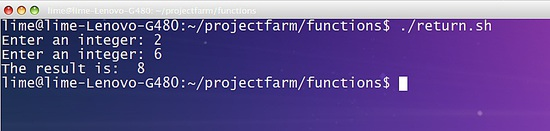The echo \$1 and echo \$2 in the last two lines of our script have no display since the interpreter does not recognize both variables because they are both local to the myfunction.

## Returning Values from Functions

Aside from creating functions and passing parameters to it, bash functions can pass the values of a function's local variable to the main routine by using the keyword return. The returned values are then stored to the default variable \$? For instance, consider the following code:

``#!/bin/bashadd(){    sum=\$((\$1+\$2))    return \$sum}read -p "Enter an integer: " int1read -p "Enter an integer: " int2add \$int1 \$int2echo "The result is: " \$?``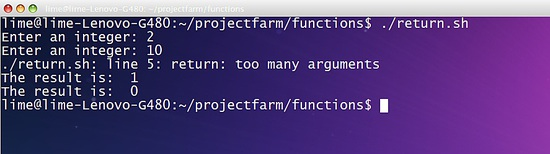In the example, we pass the parameters int1 and int2 to the add function. Next the add function processes it through the line sum=\$((\$1+\$2)). Then the value of the sum variable is passed to the main routine through the line return \$sum. By default, the values of \$sum will be stored to the default variable \$? Finally, the line echo "The result is: " \$? prints the result.

``Note: Shell scripts can only return a single value.``

Unlike other programming languages, shell scripts cannot return multiple values from a function. Let's take a look in this example:

``#!/bin/bashadd(){    sum=\$((\$1+\$2))    dif=\$((\$1-\$2))    return \$sum }read -p "Enter an integer: " int1read -p "Enter an integer: " int2add \$int1 \$int2echo "The result is: " \$?echo "The result is: " \$?``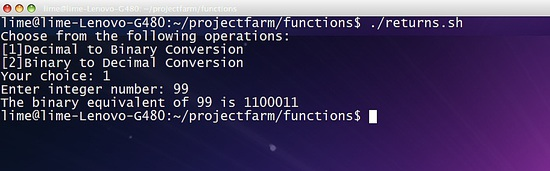## To sum it up

Let's have another example that uses functions, passes parameters to it and returns value.

``#!/bin/bash######################Author: HowtoForge ###################### clear(){    clear}bin(){    bin1=\$(echo "obase=2;\$1"|bc)    echo \$bin1}dec(){    dec1=\$(echo "ibase=2;\$1"|bc)    return \$dec1}########Main#########    printf "Choose from the following operations:\nDecimal to Binary Conversion\n"    printf "Binary to Decimal Conversion\n"    read -p "Your choice: " op    case \$op in    1)        read -p "Enter integer number: " int        bin \$int    ;;    2)        read -p "Enter binary number: " int        dec \$int        echo "The decimal equivalent of \$int is \$?"    ;;    *)        echo "Wrong Choice!"    esac``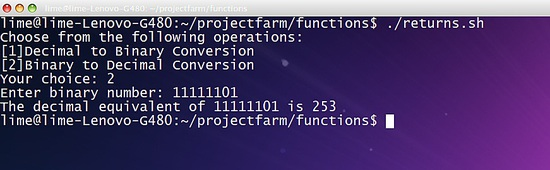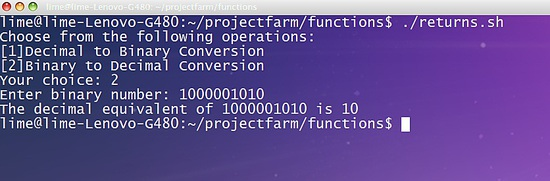The given example converts a given input to both binary or decimal value using obase and ibase command. The line \$(echo "obase=2;\$1"|bc) converts a given decimal value to binary digit and store it to bin1 variable. Next we displayed the value of \$bin1 by using echo command.

``Note: It's better to use echo directly when converting from decimal to binary because when you return command to pass a binary value, the bash converts the binary value to decimal before returning it.``

Additionally, we have converted the binary value to decimal using the command \$(echo "ibase=2;\$1"|bc).

You must also remember that the interpreter is only capable of accepting 8-bit binary digit. You will input digit that exceeds the 8-bit limit, it will generate an overflow and the most significant bit of the digit will be discarded.

The 10-bits binary digit 1000001010 returns 10 since following the 8-bit rule, the remaining 2 bits in the right side (most significant bit) will be omitted, thus, 1000001010 will become equal to 00001010 which is equal to 10. If you want an operation that accepts binary digits exceeding 8-bits then you have to create the code manually.

## Conclusion

Bash has functionalities that are very similar to programming languages to provide numerous tools to the user and make Linux systems more powerful. In this series, you have enhanced your knowledge in shell scripting through functions. Functions in shell scripts to provide modularity to the user, making scripts easier to troubleshoot and enable code reuse.

## Reference:

 American Heritage® Dictionary of the English Language, Fifth Edition. Copyright © 2011 by Houghton Mifflin Harcourt Publishing Company. Published by Houghton Mifflin Harcourt Publishing Company.view as pdf |print

## 8 Comment(s)

I like subshells. In a bash shell script, a subshell is code that is surrounded by parentheses. It runs as a separate process. You can see it in the process table. A subshell can be backgrounded & redirected like any other code, and subshells can be nested like russian dolls. This takes advantage of inheritance, as explained in 'man fork'. If you want to do 2 or more things at once in a shell script, like create/update a logfile and at the same time monitor that logfile, you will need at least one subshell.

Examples: everyone needs a timer. Zenity required. http://user.cavenet.com/rolandl/timer.txt

Custom action for your file manager: http://user.cavenet.com/rolandl/ftp-user.txt

By: Kenneth

typo on the function syntax?

syntax 1:

function function_name

{

##commands

}

syntax 2:

function_name ()

{

##commands

}

By: till

As far as I can see, the example is ok. You can define a function with and without the keyword "function" in front of the function name in the bash shell.

By: Kenneth

I'm pertaining to the use of () which should not be present on the first syntax.

By: till

Thanks, I corrected that.

By: Mike T

Can bash functions export variables from within functions?

By: thctlo

Yes it can.